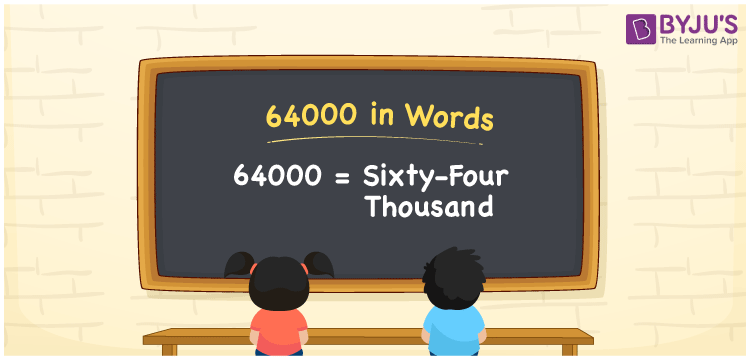# 64000 in Words

64000 in words can be written as Sixty-four thousand. The fundamental concepts in Mathematics like counting or count can be learnt efficiently here. If you buy a bike for Rs. 64000, then you can say that “I bought a bike for Sixty-four thousand Rupees”. To write numbers in words, the English alphabet is used. The numbers in words concept is explained here in a simple way to improve the conceptual knowledge of students. The 64000 can be read as “Sixty-four thousand” in English.

 64000 in words Sixty-four thousand Sixty-four thousand in Numbers 64000

## 64000 in English Words## How to Write 64000 in Words?

Students can learn about the expanded form and the place value chart of 64000. Five digits are present in the number 64000. With the help of the place value chart given below, students will be able to understand the concepts with ease.

 Ten Thousands Thousands Hundreds Tens Ones 6 4 0 0 0

64000 can be written in expanded form as:

6 x Ten Thousand + 4 x Thousand + 0 × Hundred + 0 × Ten + 0 × One

= 6 x 10000 + 4 x 1000 + 0 × 100 + 0 × 10 + 0 × 1

= 60000 + 4000

= 64000

= Sixty-four thousand

Hence, 64000 in words is written as Sixty-four thousand.

64000 is a natural number that precedes 64001 and succeeds 63999.

64000 in words – Sixty-four thousand

Is 64000 an odd number? – No

Is 64000 an even number? – Yes

Is 64000 a perfect square number? – No

Is 64000 a perfect cube number? – Yes

Is 64000 a prime number? – No

Is 64000 a composite number? – Yes

## Frequently Asked Questions on 64000 in Words

Q1

### How to write 64000 in words?

64000 can be written in words as “Sixty-four Thousand”.
Q2

### How to write Sixty-four Thousand in numbers?

Sixty-four Thousand in numbers can be written as 64000.
Q3

### Is 64000 an odd or even number?

64000 is an even number as it is completely divisible by 2.
64000/2 = 32000